## Bank UML Activity Diagram

13
0
1
publish time: 2021-03-26Captain O Captain

Bank Activity Diagrams are used to illustrate the flow of control in a system and refer to the steps involved in the execution of a use case. A bank UML activity diagram focuses on the condition of flow and the sequence in which it happens. UML models basically three types of diagrams: structure diagrams, interaction diagrams, and behavior diagrams. As shown in the image, the bank UML activity diagram is very similar to a flowchart and is used in business and process modeling where their primary use is to depict the dynamic aspects of a system. Online tools like EdrawMax Online lets you create wonderful Bank UML Activity diagrams, as it provides several icons, background images, and symbols that are required to create a conceptually correct activity diagram.

See More Related TemplatesThis is an example of a UML diagram about adding events to the calendar.
UML Diagram - Add event to calendar
23
1
0easy diagrams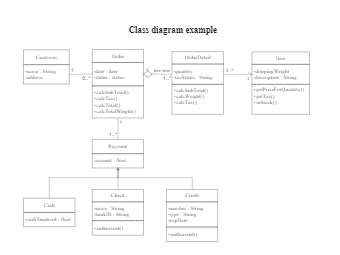This is an example of a uml class diagram.
Class diagram example
187
5
4easy diagrams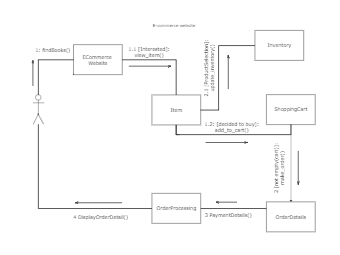This is an example of a UML collaboration diagram.
ecommerce website - UML diagram
20
2
0easy diagrams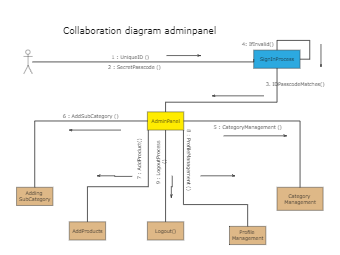10
1
0easy diagrams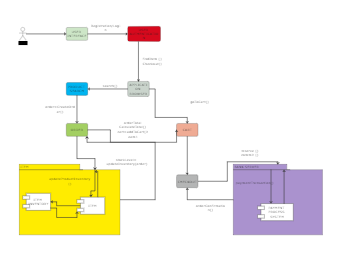This is an example of a UML communication diagram.
Communication diagram example
11
1
1easy diagrams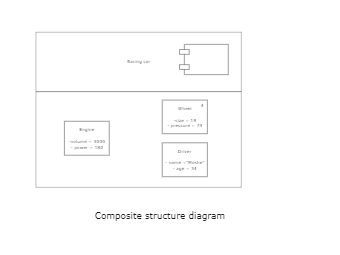This is an example of a composite structure diagram.
Composite structure diagram
3
0
0easy diagrams